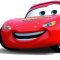## Multiples of 3 or 7 in C++Hafeezul Kareem

Updated on 25-Oct-2021 05:40:28

Given a number n, we need to find the count of multiples of 3 or 7 till n. Let's see an example.Input100Output43There are total of 43 multiples of 3 or 7 till 100.AlgorithmInitialise the number n.Initialise the count to 0.Write a loop that iterates from 3 to n.Increment the count ... Read More

## Move last element to front of a given Linked List in C++Hafeezul Kareem

Updated on 25-Oct-2021 05:21:53

Given a linked list, we have to move the last element to the front. Let's see an example.Input1 -> 2 -> 3 -> 4 -> 5 -> NULLOutput5 -> 1 -> 2 -> 3 -> 4 -> NULLAlgorithmInitialise the linked list.Return if the linked list is empty or it has ... Read More

## Move first element to end of a given Linked List in C++Hafeezul Kareem

Updated on 25-Oct-2021 05:13:06

<Given a linked list, we have to move the first element to the end. Let's see an example.Input1 -> 2 -> 3 -> 4 -> 5 -> NULLOutput2 -> 3 -> 4 -> 5 -> 1 -> NULL AlgorithmInitialise the linked list.Return if the linked list is empty or it ... Read More

## Move all zeros to the front of the linked list in C++Hafeezul Kareem

Updated on 25-Oct-2021 05:02:21

Given a linked list with random integers and zeroes. We have to move all the zeroes to the front of the linked list. Let's see an example.Input3 -> 0 -> 1-> 0 -> 0 -> 1 -> 0 -> 0 -> 3 -> NULLOutput0->0->0->0->0->3->1->1->3->NULL AlgorithmInitialise the linked list.Return if the ... Read More

## Move all zeros to start and ones to end in an Array of random integers in C++Hafeezul Kareem

Updated on 25-Oct-2021 04:49:34

In this tutorial, we are going to write a program that moves all zeroes to front and ones to end of the array.Given an array with zeroes and ones along with random integers. We have to move all the zeroes to start and ones to the end of the array. ... Read More

## Move all zeroes to end of array in C++Hafeezul Kareem

Updated on 25-Oct-2021 04:44:33

Given array with multiple zeroes in it. We have to move all the zeroes in the array to the end. Let's see an example.Inputarr = [4, 5, 0, 3, 2, 0, 0, 0, 5, 0, 1]Output4 5 3 2 5 1 0 0 0 0 0AlgorithmInitialise the array.Initialise an index ... Read More

## Motzkin number in C++Hafeezul Kareem

Updated on 25-Oct-2021 04:40:19

The Motzkin number series starts with 1, 1, 4, 9, etc.., We can get the generalised nth term with the sequence. The Motzkin number sequence is as follows.a0 = 1a1 = 1a2 = 4a3 = 9an = ((2 * n + 1)/ n + 2) * M(n-1) +((3 * n ... Read More

## Most frequent element in an array in C++Hafeezul Kareem

Updated on 25-Oct-2021 04:35:35

We are given a array and we need to find the most frequent element from it. Let's see an example.Inputarr = [1, 2, 3, 3, 2, 2, 1, 1, 2, 3, 4]Output2In the above array, 2 occurs 4 times which is most frequent than any other in the array.Algorithm - ... Read More

## Next smallest prime palindrome in C++Hafeezul Kareem

Updated on 25-Oct-2021 04:27:23

We are given a number N. We need to find the prime palindrome that is greater than N. Let's see an example.InputN = 10Output11AlgorithmInitialise the number N.Write a function to check whether the given number is prime or not.Write a function to check whether the given number is palindrome.Write a ... Read More

## Next Smaller Element in C++Hafeezul Kareem

Updated on 25-Oct-2021 04:07:58

The next smaller element is the element that is the first smaller element after it. Let's see an example.arr = [1, 2, 3, 5, 4]The next smaller element for 5 is 4 and the next smaller element for elements 1, 2, 3 is -1 as there is no smaller element ... Read More